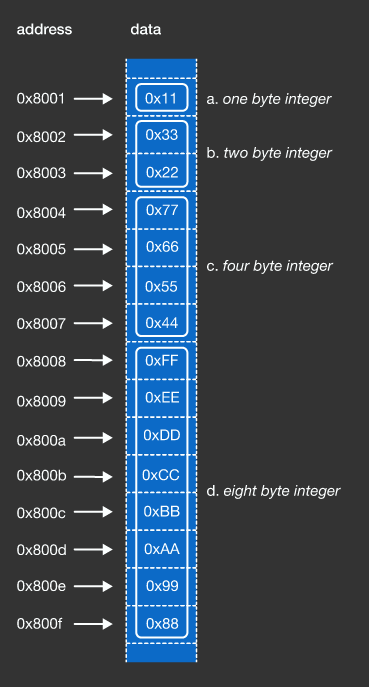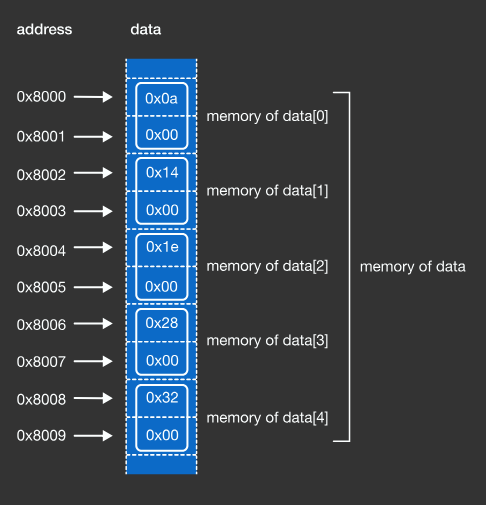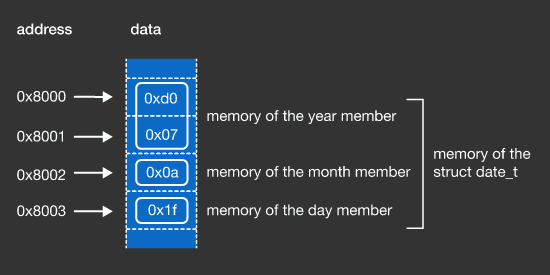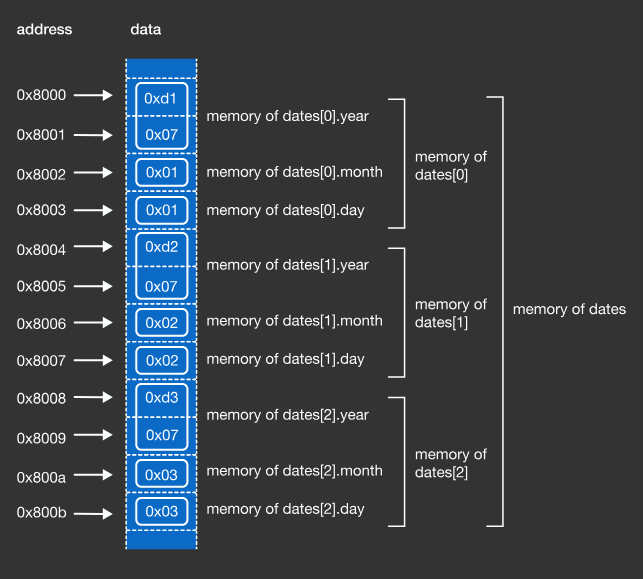### Computer Memory

In this quiz, we are going to see how variables in programs get placed in memory. We will use the programming language C for explanation. There are a few data types that represent integers in C, ie. char, short, int, and long. The size of each data type depends on the compiler and computer architecture, but we will assume the following sizes here:

char … 1 byte
short … 2 bytes
int … 4 bytes
long … 8 bytes

When programming, we need to decide on the size of the integer to use. We often use int, the default type, which has 4 bytes and can hold integers in the range about -2 billion to +2 billion, and this is enough for most cases. We use the other data types for situations where we want larger or smaller integers. For example, we use long when we need a variable that can hold numbers that are larger than 2 billion. We may use char or short when we want to save memory.

Question:
What is the total amount of memory, in bytes, needed when allocating the following four variables?

 1 2 3 4 char a; short b; int c; long d; 

# Memory Layout

Let’s consider the memory of the following variables:

 1 2 3 4 char a = 0x11; short b = 0x2233; int c = 0x44556677; long d = 0x8899AABBCCDDEEFF; 

On an example environment, the memory layout may look like the following diagram:In this example, the order of variables defined in the source code and the order of the data stored in memory matched each other. Furthermore, the variables were placed next to each other in memory without any padding in between. However, in actual situations, the compiler may reorder variable allocation and add padding for optimization.

# Memory Layout

We often use arrays in our programs. This is a data type to describe a collection of elements, where each element can be selected by an index. For example, an array that has five short integers, can be described as follows:

short data = {10, 20, 30, 40, 50};

The memory layout of an array is a continuous chunk of memory, the size of which is $\text{sizeof}(\text{element}) \times \text{length}$. What is the total amount of memory needed for this array, in bytes?

# Memory Layout

short data = {10, 20, 30, 40, 50};

Let's suppose that the memory layout of this array looks like the following diagram:The array data is allocated from address 0x8000 to address 0x8009. When we say “the address of XYZ”, we mean “the address of the first byte of XYZ”. Therefore, the address of array data is 0x8000, and the address of element data is also 0x8000.

# Memory Layout

Since the address of the array is the same as the address of the element at index $0$, the address of the element at index $N$ is $\text{head} + \text{sizeof}(\text{element}) \times \text{N}$, where $\text{head}$ is the address of the array. Given the following array of integers:

int data = {0, 100, 200, 300, … };

Let 0x9000 be the the address of the array data. What is the address of the element that contains the value 1500?

# Memory Layout

A struct is a composite data type that groups a set of variables together. Other programming languages have similar concepts such as classes in Java or records in Pascal. For example, it is convenient to group year, month, and day together to represent a date. We can define struct date_t in the following way:

 1 2 3 4 5 struct date_t { short year; char month; char day; }; 

Once defined, we can allocate a struct date_t and use the fields in the following way to represent the date October 31, 2010.

 1 2 3 4 5 struct date_t d1; d1.year = 2010; d1.month = 10; d1.day = 31; 

What is the total amount of memory needed for one struct date_t in bytes?

# Memory Layout

 1 2 3 4 5 struct date_t { short year; char month; char day; }; 

The memory layout of one struct date_t will look like the following diagram:The beginning address of the struct date_t and its first member are the same, and all members are placed in the order of the source code. The compiler may insert padding between members for optimization in practical situations, but we will assume that no padding was added here. The beginning address of the struct date_t is the base address. The addresses of the members are calculated as $\text{base} + \text{offset}$, where $\text{offset}$ is the total size of the prior members in bytes. For example, the offset for day is 3, since that is the total size of the prior members year and month. The offset for year is 0 since there are no members prior to it.

# Memory Layout

Arrays can hold struct elements as well as primitive types. For the following code, let 0x7000 be the base address of the dates array. What is the address of the struct member that contains the value 3?

  1 2 3 4 5 6 7 8 9 10 11 12 13 14 15 16 17 18 19 struct date_t { short year; char month; char day; }; struct date_t dates; dates.year = 2001; dates.month = 1; dates.day = 10; dates.year = 2002; dates.month = 2; dates.day = 20; dates.year = 2003; dates.month = 3; // this member dates.day = 30; 

# Memory Layout

The memory layout of the dates array will look like the following:# Memory Layout

×